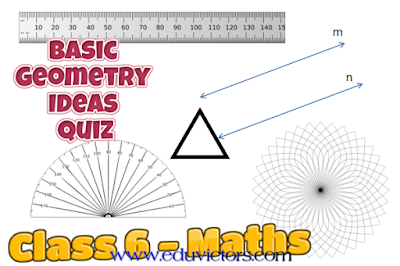## Pages

Showing posts with label geometry. Show all posts
Showing posts with label geometry. Show all posts

## Basic Geometry Ideas - Quiz

Class 6 - Mathematics

Q1: Three or more lines which pass through same point are called

(a) intersecting lines
(b) parallel lines
(c) perpendicular lines
(d) concurrent lines.

Q2: Geometrical figure which has no dimension is

(a) line
(b) plane
(c) line segment
(d) point.

Q3: The lines which do not intersect and have equal distance between them are called:

(a) parallel lines
(b) perpendicular lines
(c) intersecting lines
(d) straight lines